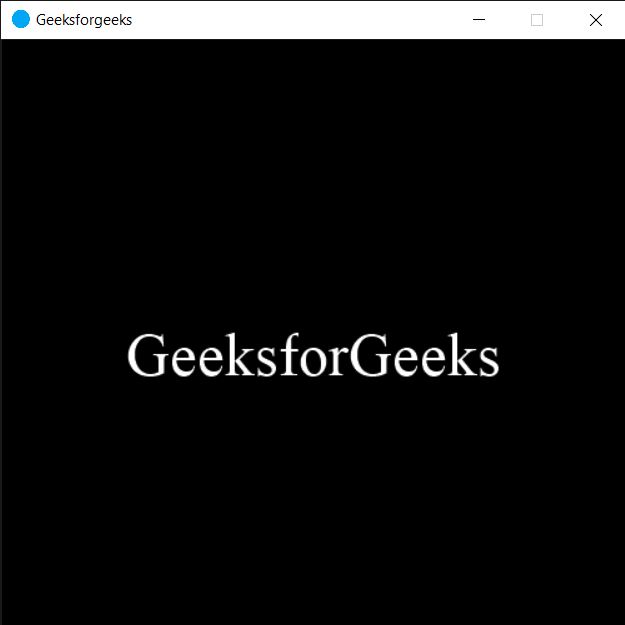# PYGLET – Getting Window Caption

• Last Updated : 22 Sep, 2020

In this article we will see how we can get the window caption in PYGLET module in python. Pyglet is easy to use but powerful library for developing visually rich GUI applications like games, multimedia etc. A window is a “heavyweight” object occupying operating system resources. Windows may appear as floating regions or can be set to fill an entire screen (fullscreen). Window caption is basically the title of the window, we can set caption while creating a window although we can change the title any time, this caption appear in the taskbar of the window. We use `set_caption` method to set the caption.

We can create a window with the help of command given below

``` pyglet.window.Window(width, height, title)
```

In order to create window we use `caption` attribute with the window object

Syntax : window.caption

Argument : It takes no argument

Return : It returns string

Below is the implementation

 `# importing pyglet module``import` `pyglet``import` `pyglet.window.key`` ` `# width of window``width ``=` `500`` ` `# height of window``height ``=` `500`` ` `# caption i.e title of the window``title ``=` `"Geeksforgeeks"`` ` `# creating a window``window ``=` `pyglet.window.Window(width, height, title)`` ` `# text ``text ``=` `"GeeksforGeeks"`` ` `# creating a label with font = times roman``# font size = 36``# aligning it to the centre``label ``=` `pyglet.text.Label(text,``                          ``font_name ``=``'Times New Roman'``,``                          ``font_size ``=` `36``,``                          ``x ``=` `window.width``/``/``2``, y ``=` `window.height``/``/``2``,``                          ``anchor_x ``=``'center'``, anchor_y ``=``'center'``)`` ` `new_label ``=` `pyglet.text.Label(text,``                          ``font_name ``=``'Times New Roman'``,``                          ``font_size ``=` `10``,``                          ``x ``=` `25``, y ``=` `25``)`` ` `# on draw event``@window``.event``def` `on_draw():    `` ` `     ` `    ``# clearing the window``    ``window.clear()``     ` `    ``# drawing the label on the window``    ``label.draw()`` ` `     ` `# key press event    ``@window``.event``def` `on_key_press(symbol, modifier):`` ` `    ``# key "C" get press``    ``if` `symbol ``=``=` `pyglet.window.key.C:``         ` `        ``# closing the window``        ``window.close()``     ` ` ` `# image for icon``img ``=` `image ``=` `pyglet.resource.image(``"logo.png"``)`` ` `# setting image as icon``window.set_icon(img)`` ` `# getting window caption``value ``=` `window.caption`` ` `# printing the value``print``(``"Caption : "``, end ``=``"")``print``(value)`` ` `                ` `# start running the application``pyglet.app.run()`

Output :```Caption : Geeksforgeeks
```

My Personal Notes arrow_drop_up